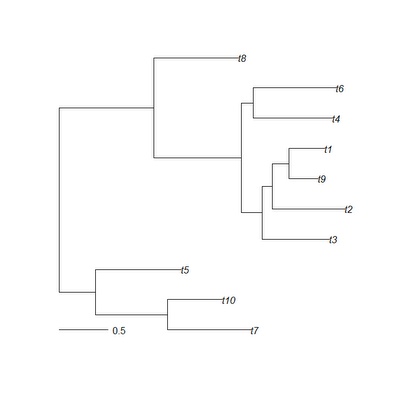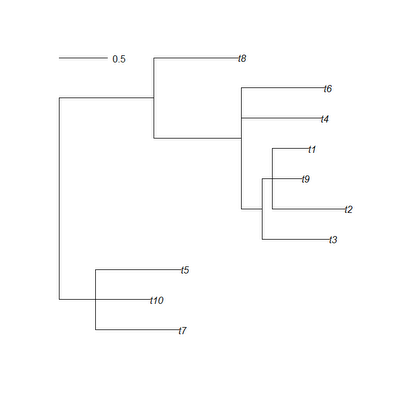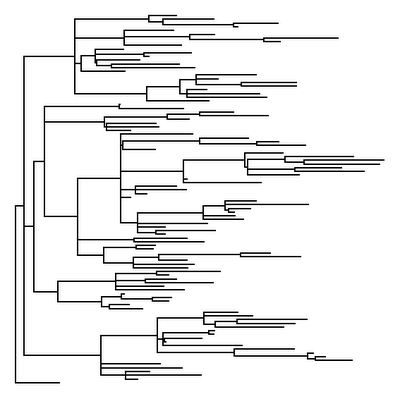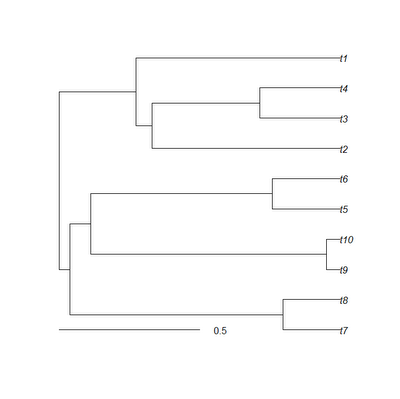## Saturday, March 17, 2012

### Trick to get the mean height of internal nodes

Here's a quick trick I came up with while working on something else today that gives the mean height of internal nodes (above the root) on a phylogenetic tree.

First, some quick background. The phytools function nodeHeights computes the height above the root for all the nodes and tips of the tree, and it returns them in a matrix of dimensions tree\$edge [the matrix containing the parent (left column) and daughter (right column) node numbers of your tree]. So, for instance:

> require(phytools)
> tree<-rtree(10)> H<-nodeHeights(tree)
> H
[,1]      [,2]
[1,] 0.0000000 0.3767669
[2,] 0.3767669 1.1140877
[3,] 1.1140877 1.9673950
[4,] 1.1140877 1.6751804
[5,] 0.3767669 1.2550270
[6,] 0.0000000 0.9705378
[7,] 0.9705378 1.8688737
[8,] 1.8688737 2.0820751
[9,] 2.0820751 2.7701772
[10,] 2.0820751 2.1866002
[11,] 2.1866002 2.9329200
[12,] 2.1866002 2.3545078
[13,] 2.3545078 2.6565813
[14,] 2.3545078 2.7237393
[15,] 1.8688737 1.9883786
[16,] 1.9883786 2.8042467
[17,] 1.9883786 2.8374805
[18,] 0.9705378 1.8377918

It's clear from a casual inspection of the matrix that each parent node height (in the right column) is represented twice and only twice. Thus, if we exclude the root node (zero height), we can just take the mean of H[,1].

> hbar1<-mean(sort(H[,1])[3:nrow(H)])
> hbar1
 1.617728

The only problem with this is that it will not work for trees in which some nodes have more than two descendants (i.e., multifurcating trees). Instead, we can do the following:

> hbar2<-mean(H[sapply(length(tree\$tip)+2:tree\$Nnode,match, tree\$edge[,1]),1])
> hbar2
 1.617728

This works by matching all internal node numbers, excluding the root, to the first column of tree\$edge, and then pulling only those elements out of H[,1]. Importantly, the base function match identifies the index of only the first instance of an item in a vector. (The function which can be used to pull the indices of multiple matching items from a vector.)

To see how this matters, let's collapse some of the nodes of our tree into multifurcations.

> tree\$edge.length[c(2,12,15)]<-0
> tree<-di2multi(tree)> hbar1<-mean(sort(H[,1])[3:nrow(H)])
> hbar1
 1.492458
> hbar2<-mean(H[sapply(length(tree\$tip)+2:tree\$Nnode,match, tree\$edge[,1]),1])
> hbar2
 1.496971

(OK, so it is a very small effect in this case, but it could be significant. That's what I get for not running my example all the way through before posting it.)

## Friday, March 16, 2012

### Phylogenetic signal with K and λ

A recent Google search string that led a reader to my blog was the following:

different phylogenetic signal with K vs lambda.

Phylogenetic signal is generally recognized to be the tendency of related species to resemble one another; and Blomberg et al.'s (2003) K and Pagel's (1999) λ are two quantitative measures of this pattern. The metrics are quite different from one another. λ is a scaling parameter for the correlations between species, relative to the correlation expected under Brownian evolution. K is a scaled ratio of the variance among species over the contrasts variance (the latter of which will be low if phylogenetic signal is high). λ has a nice natural scale between zero (no correlation between species) and 1.0 (correlation between species equal to the Brownian expectation). λ itself is not a correlation, but a scaling factor for a correlation, so λ>1.0 is theoretically possible. However, depending on the structure of the tree, λ>>1.0 is usually not defined. K, a variance ratio, is rescaled by dividing by the Brownian motion expectation. This gives it the property of having an expected value of 1.0 under Brownian evolution, but K for empirical and simulated datasets can sometimes be >>1.0. Since they measure the qualitative tendency towards similarity of relatives in entirely different ways, we have no real expectation that they will be numerically equivalent - except (by design) under Brownian motion evolution.

So, accepting that λ & K are different measures of phylogenetic signal, we might reasonably ask - do statistical tests based on each measure tend to find significant phylogenetic signal for the same datasets? One way to crudely test this would be simulate datasets for various generating λ and then ask if significant tests based on λ and K tend to be associated.

require(phytools); require(geiger)
nrep<-1000
P.K<-P.l<-l<-vector()
for(i in 1:nrep){
tree<-pbtree(n=50)
l[i]<-runif(n=1)
x<-fastBM(lambdaTree(tree,l[i]))
K<-phylosig(tree,x,test=T)
lambda<-phylosig(tree,x,method="lambda",test=T)
P.K[i]<-K\$P
P.l[i]<-lambda\$P
}

Compute the fraction of significant tests using K & λ, and the fraction expected to be the same (either both significant or both non-significant) if they are independent (this we compute as the product of the preceding fractions plus the product of 1 minus each fraction):

> pK<-mean(P.K<=0.05); pK
 0.417
> pL<-mean(P.l<=0.05); pL
 0.514
> pK*pL+(1-pK)*(1-pL)
 0.497676

Now, the fraction of times that tests on λ & K yield the same result (i.e., either both significant or both non-significant):

> mean(((P.K<=0.05)==(P.l<=0.05)))
 0.765

Obviously, there is some relation between the tests of the two metrics, but it is not extremely strong. Let's also ask if the average generating value of λ is higher when either or both λ and K produce a significant result:

> # first lambda
> mean(l[P.l<=0.05]) # lambda significant
 0.6671113
> mean(l[P.l>0.05]) # not significant
 0.2884
> # then K
> mean(l[P.K<=0.05]) # K significant
 0.6842788
> mean(l[P.K>0.05]) # not significant
 0.339131
> # then P & lambda
> mean(l[(P.K<=0.05)*(P.l<=0.05)==1]) # lambda & K significant
 0.7352098
> mean(l[(P.K<=0.05)*(P.l<=0.05)==0]) # neither significant
 0.3484733

## Thursday, March 15, 2012

### Contrasts regression with multifurcations

An R phylogenetics user just emailed me about a post I wrote (archived here) describing how to resolve multifurcations before calculating contrasts for PIC regression. I just wanted to take a couple of moments to elaborate on this issue.

The contrasts algorithm (Felsenstein 1985) requires a fully dichotomous (i.e. fully bifurcating) phylogenetic tree with branch lengths. By computing the differences between tip & node states, the contrasts algorithm creates a transformed dataset which (under a Brownian model of evolution) is free from phylogenetic dependence.

However, if your phylogeny is not fully dichotomous, it can be rendered dichotomous by arbitrarily resolving all polytomies through the addition of one or more internal branches of length 0.0; after which contrasts can be calculated as normal. Since every trifurcation can be resolved in at least three ways (and higher order multifurcations in even more different ways), we may have many options in how we choose to resolve our non-dichotomous tree. For instance, if our tree is fully bifurcating except at two nodes which are each trifurcations, we have 3 × 3 = 9 different ways of rendering our tree dichotomous via the addition of internal zero length branches.

Luckily, it turns out not to matter how we resolve the tree: statistical analysis of the contrasts transformed data is unaffected. To prove this to ourselves, let's look at two different examples:

> require(phytools)
> # first let's create a random tree with polytomies
> tree<-rtree(n=100)
> # pick nodes to collapse
> nodes<-100+sample(2:tree\$Nnode)[1:30]
> # match the nodes to tree\$edge & set to zero
> tree\$edge.length[apply(apply(as.matrix(nodes),1,"==", as.matrix(tree\$edge[,2])),2,which)]<-0
> # collapse 0 length branches to multifurcations
> tree<-di2multi(tree)
> plotTree(tree,pts=F,ftype="off")Close inspection of this tree reveals that it has many multifurcations, and this is corroborated by the fact that the tree can be seen to have 30 fewer nodes (just as we'd hoped) than the number expected for a fully dichotomous 100 taxon tree:

> tree\$Nnode
 69

The next thing we'll do is simulate under an arbitrary linear model, here y = 1.5 + 0.75x + ε, but we will do so in such a way so that the data are phylogenetically autocorrelated:

> x<-fastBM(tree) # explanatory variable
> y<-1.5+0.75*x+fastBM(tree,sig2=0.2) # arbitrary linear model

OK, now let's resolve the tree randomly, compute contrasts, and fit a bivariate regression model to the contrasts:

> rt1<-multi2di(tree,random=TRUE)
> pic.x<-pic(x,rt1)
> pic.y<-pic(y,rt1)
> lm(pic.y~pic.x-1) # fits the regression model without an intercept

Call:
lm(formula = pic.y ~ pic.x - 1)

Coefficients:
pic.x
0.7761

To show that we get the same result from contrasts regression regardless of the random resolution that we use, let's try a different random resolution and fit the regression model again:

> rt2<-multi2di(tree,random=TRUE)
> pic.x<-pic(x,rt2)
> pic.y<-pic(y,rt2)
> lm(pic.y~pic.x-1)

Call:
lm(formula = pic.y ~ pic.x - 1)

Coefficients:
pic.x
0.7761

To assure ourselves that this is not just magical good luck at having obtained the same resolution in each case we can compute:

> all.equal.phylo(rt1,rt2)
 FALSE

and see that it is FALSE.

We have to avoid the following tempting shortcut:

> lm(pic(y,multi2di(tree))~pic(x,multi2di(tree))-1)

Call:
lm(formula = pic(y, multi2di(tree)) ~ pic(x, multi2di(tree)) -
1)

Coefficients:
pic(x, multi2di(tree))
-0.01451

or variants thereof, because in this case different random resolutions are used for x & y, which is not permitted. However, now knowing that the specific choice of resolution doesn't matter (so long as it is the same for dependent & independent variables), we can also see that the following will work:

> lm(pic(y,multi2di(tree,random=FALSE))~pic(x,multi2di(tree, random=FALSE))-1)

Call:
lm(formula = pic(y, multi2di(tree, random = FALSE)) ~ pic(x,
multi2di(tree, random = FALSE)) - 1)

Coefficients:
pic(x, multi2di(tree, random = FALSE))
0.7761

In this case, multi2di(...,random=FALSE) polytomies are not resolved randomly, but in the order they appear in the tree (which appears to be right-to-left in the input Newick string).

## Wednesday, March 7, 2012

### Testing whether Pagel's lambda is different from 1.0

Is there an easy way to use phylosig to test whether the fitted value of λ is significantly different from 1?

Indeed, this is possible to do using fitContinuous in the geiger package, as many readers probably realize. It is also possible to do this with functions entirely in the phytools package. This is how:

# assuming phylogeny in 'tree' and data in 'x'
mtree<-tree
mtree\$mapped.edge<-matrix(mtree\$edge.length,nrow(tree\$edge),1)
colnames(mtree\$mapped.edge)<-"1"
resBM<-brownie.lite(mtree,x)
resLambda<-phylosig(tree,x,method="lambda")
LR<-2*(resLambda\$logL-resBM\$logL1)
P<-pchisq(LR,df=1,lower.tail=F)

That's it!

## Monday, March 5, 2012

### Function to model selection at a biallelic locus

This is not for phytools, but I am teaching evolutionary biology to undergrads this semester. We are presently studying population genetics and for class tomorrow I decided to write an R function to illustrate selection at one locus. The function takes a starting allele frequency and fitnesses for each of the three genotypes as input, and then it can plot various things: the frequency of A or a over time; the mean fitness through time; and the population mean fitness as a function of p. The function is as follows:

selection<-function(p0,w,time=1000,show="p",pause=0){
p<-wbar<-vector(); p<-p0
wbar<-p^2*w+2*p*(1-p)*w+(1-p)^2*w
for(i in 2:time){
p[i]<-p[i-1]
p[i]<-(p[i]^2*w+p[i]*(1-p[i])*w)/wbar[i-1]
wbar[i]<-p[i]^2*w+2*p[i]*(1-p[i])*w+(1-p[i])^2*w
if(show=="p")
plot(1:i,p,type="l",xlim=c(0,time),ylim=c(0,1),
xlab="time",main="frequency of A")
else if(show=="q")
plot(1:i,1-p,type="l",xlim=c(0,time),ylim=c(0,1),xlab="time",
ylab="q",main="frequency of a")
else if(show=="fitness")
plot(1:i,wbar/max(w),type="l",xlim=c(0,time),ylim=c(0,1),
xlab="time",main="mean fitness")
else if(show=="surface")
break
else {
message("not a recognized option")
break
}
Sys.sleep(pause)
}
if(show=="surface"){
p<-0:100/100
wbar<-p^2*w+2*p*(1-p)*w+(1-p)^2*w
plot(p,wbar,type="l",ylim=c(0,1),main="mean fitness")
}

Here is an example of a run to illustrate selection on A in which the fitness advantage of the A allele is recessive.

## Saturday, March 3, 2012

### New version of treeSlice

The phytools function treeSlice cuts a phylogeny at a particular height above the root and returns all non-trivial subtrees (that is, subtrees with two or more taxa). A user requested that I add the capacity to return trivial as well as non-trivial subtrees. This is straightforward, but it cannot be done using the ape function extract.clade, because that only permits the extraction of trees with two or more tips. Instead, for subtrees with only one tip we create the "phylo" object directly. Here is how we do it:

for(i in 1:length(nodes)){
if(nodes[i]>length(tree\$tip)){
trees[[i]]\$root.edge<-H[which(tree\$edge[,2]==nodes[i]),2] -slice
} else {
z<-list(edge=matrix(c(2,1),1,2), edge.length=H[which(tree\$edge[,2]==nodes[i]),2]-slice, tip.label=tree\$tip.label[nodes[i]],Nnode=1L)
class(z)<-"phylo"; trees[[i]]<-z
}
}

Seems to work fine. The default performance of treeSlice is to return only non-trivial subtrees. To return trivial subtrees, we set the new argument trivial=TRUE. So, for instance:

> set.seed(1)
> tree<-pbtree(n=10,scale=1)> trees<-treeSlice(tree,0.5,trivial=T)
> str(trees)
Class "multiPhylo"
List of 6
\$ :List of 5
..\$ edge       : num [1:2, 1:2] 3 3 1 2
..\$ edge.length: num [1:2] 0.204 0.204
..\$ tip.label  : chr [1:2] "t7" "t8"
..\$ Nnode      : int 1
..\$ root.edge  : num 0.296
..- attr(*, "class")= chr "phylo"